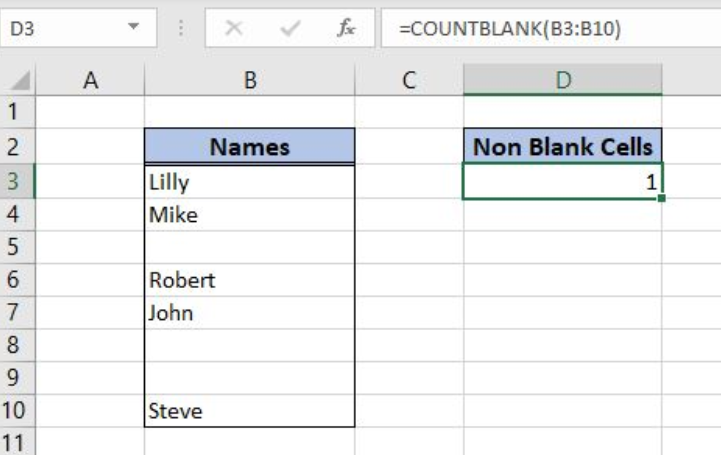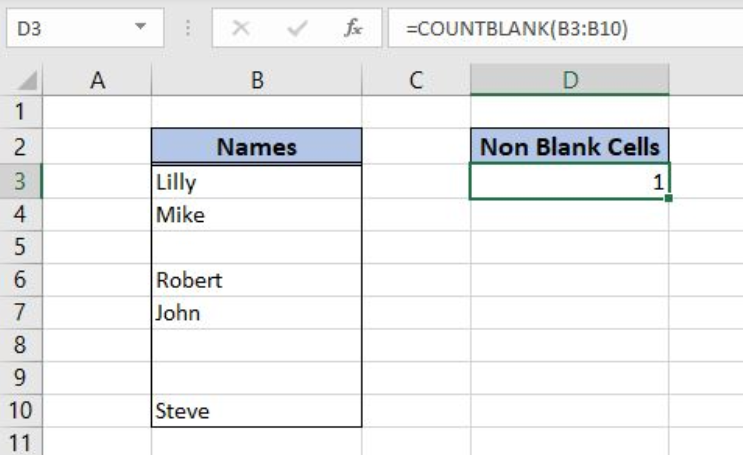Get instant live expert help with Excel or Google Sheets“My Excelchat expert helped me in less than 20 minutes, saving me what would have been 5 hours of work!”

#### Post your problem and you’ll get expert help in seconds.

Your message must be at least 40 characters
Our professional experts are available now. Your privacy is guaranteed.

# Excel COUNTBLANK Function

Excel allows a user to count all cells from a range that are blank, by using the COUNTBLANK function.  The function takes in count texts, numbers, spaces, results of other function, etc. This step by step tutorial will assist all levels of Excel users in counting blank cells in a range.Figure 1. The result of the COUNTBLANK function

## Syntax of the COUNTBLANK Formula

The generic formula for the COUNTBLANK function is:

`=COUNTBLANK(range)`

The parameter of the COUNTBLANK function is:

• range – a range of cells where we want to count non-blank cells.

## Counting Blank Cells Using the COUNTBLANK Function

In our example, we want to count all blank cells in the range B3:B10. In the cell B8, we put a space. In the cell B9, there is the formula which returns a space as a result. The formula looks like:

`=COUNTBLANK(B3:B10)`

The parameter range is B3:B10, while in the cell D3 we want to get a result of the COUNTBLANK function.

To apply the COUNTBLANK function, we need to follow these steps:

• Select cell D3 and click on it
• Insert the formula: `=COUNTBLANK(B3:B10)`
• Press enterFigure 2. Using the COUNTBLANK function to count non-blank cells in the range

There is only 1 blank cell in the range, as B8 and B9 contain a space. Because of that, the result of the function in the cell D3 is 1.

## Notes

If the cell contains an empty text as a result of the function, the COUNTBLANK will take it in the count.

Most of the time, the problem you will need to solve will be more complex than a simple application of a formula or function. If you want to save hours of research and frustration, try our live Excelchat service! Our Excel Experts are available 24/7 to answer any Excel question you may have. We guarantee a connection within 30 seconds and a customized solution within 20 minutes.

### Did this post not answer your question? Get a solution from connecting with the expert.Another blog reader asked this question today on Excelchat:
Solution examplesi need a formula to give me a total of cells with data the ignore the data itself.... for example I have 20 cells with date, but within the range there are 5 cells with no data. I need formula that will tell me the end sum is 15 that way if I sort the data the total numbers of cells with data will not change
Solved by F. Y. in 9 minsFormula help: =COUNTBLANK(offset(Inglewood!C\$2,,MATCH(D3,Inglewood!\$C\$1:\$N\$1,0)-1,counta(Inglewood!\$A:\$A)-1)) This is helping me have conditional formatting based on empty cells from another tab. I want to change the range of data that is being seen. (i.e. instead of C2:C47 I want it to be C11:C47)
Solved by V. U. in 31 mins## Subscribe to Excelchat.coAnother blog reader asked this question today on Excelchat: